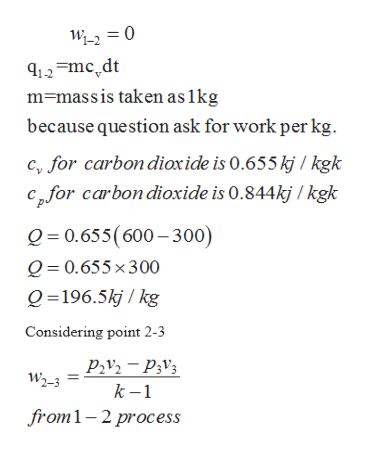# Carbon Dioxide is contained in a piston-cylinder assembly and undergoes a cycle made of the fol- lowing processes: • Process 1-2: Constant volume from 1 bar, 300 K to 600 K • Process 2–3: Polytropic expansion with n=k until P3 = P1 • Process 3-1: Isobaric compression (a) Sketch the cycle on p-v and T-v coordinates (b) Determine the work and heat transfer in each process, in kJ/kg (c) Determine the type of cycle that this is. If it is a power cycle, compute the thermal efficiency. Otherwise, compute the coefficient of performance for a heat pump cycle.

Question
38 views

Carbon Dioxide is contained in a piston-cylinder assembly and undergoes a cycle made of the fol- lowing processes: • Process 1-2: Constant volume from 1 bar, 300 K to 600 K • Process 2–3: Polytropic expansion with n=k until P3 = P1 • Process 3-1: Isobaric compression (a) Sketch the cycle on p-v and T-v coordinates (b) Determine the work and heat transfer in each process, in kJ/kg (c) Determine the type of cycle that this is. If it is a power cycle, compute the thermal efficiency. Otherwise, compute the coefficient of performance for a heat pump cycle.

check_circle

Step 1

Given data as per question

Step 2

We know that work done in constant volume is zero.

Step 3help_outlineImage TranscriptioncloseWi-2 0 12mcdt m massis taken as 1kg because question ask for work per kg c, for carbon dioxide is 0.655 kj / kgk cpfor carbon dioxide is 0.844kj / kgk Q 0.655(600-300) Q 0.655 x300 Q 196.5kj/ kg Considering point 2-3 P2v2-P3v3 W2-3 k -1 from1-2 process fullscreen

### Want to see the full answer?

See Solution

#### Want to see this answer and more?

Solutions are written by subject experts who are available 24/7. Questions are typically answered within 1 hour.*

See Solution
*Response times may vary by subject and question.
Tagged in

### Thermodynamics